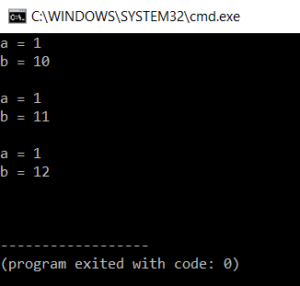# How To Implement Static Variable In C?How To Implement Static Variable In C?

Static variables are known to retain the value even after they exit the scope. They retain their value and are not initialized again in the new scope. This article will help you explore Static variable in C.

So let us get started then,

## Static Variable In C

The keyword static is used while declaring a static variable.

Syntax:

`static Data_type Var_name = Var_value;`

Example:

`static int abc=80;`

The static variable is kept in the memory till the end of the program, whereas a normal variable is destroyed when a function is over.

Moving on with this static variable in C article

Static Variables Inside the Function
The static variable inside the function holds the value not only till the end of the function block but till the end of the entire program.

Consider the code to display static keywords function,

```#include<stdio.h>
void fun();
int main()
{
fun();
fun();
fun();
return 0;
}
void fun()
{
int a = 1;
static int b = 10;
printf("a = %dn", a);
printf("b = %dnn", b);
a++;
b++;
}
```

OutputExplanation

In the above code, a function called fun is called. Inside the function block, there is a normal variable a which is initialized to 1 and a static variable b that is initialized to 10. Print the value of a and b and then increment a and b.

It can be seen that the normal variable value remains the same in all three calls but the value of the static variable keeps increasing from 10 to 11 and 12. This is because the static variable retains the value after the increment even when the function block ends.

Moving on with this static variable in C article,

Some Features of Static Variables

• Data segments are used to allocate a static variable and not stack segments.
• Static variables by default have some values with which it is initialized if not explicitly initialized.

#### By default

For pointers, it is assigned to the null pointer.

For arithmetic value, it is assigned to zero.

Consider the code,

```#include <stdio.h>
int main()
{
static int a;
printf("%d ", a);
}
```

Output:In the code, we do not initialize the value of a but by default, it is assigned to 0. Initialization of static variables is done using Constant literals

Moving on with this static variable in C article

Consider the code,

```#include<stdio.h>
int a=10,b=10;
int sum()
{
return a+b;
}
int main()
{
static int i = sum();
printf(" value of i = %d", i);
return 0;
}
```

OutputWe get an error when initialization of the variable is done using a function.

• Global variables can also be made static.

This is how the static variable is used in c programming. A static keyword can also be used for functions as well.

With this we come to the end of this blog on ‘Static Variable In C’. I hope you found this informative and helpful, stay tuned for more tutorials on similar topics.You may also checkout our training program to get in-depth knowledge on jQuery along with its various applications, you can enroll here for live online training with 24/7 support and lifetime access.Implement the above code with different strings and modifications. Now, we have a good understanding of all key concepts related to the pointer.

Got a question for us? Mention them in the comments section of  this blog and we will get back to you.